# Water flows from a large 4m detention basin (100m by 50m) along a 200mm diameter pipe for a distance of 100m at a slope...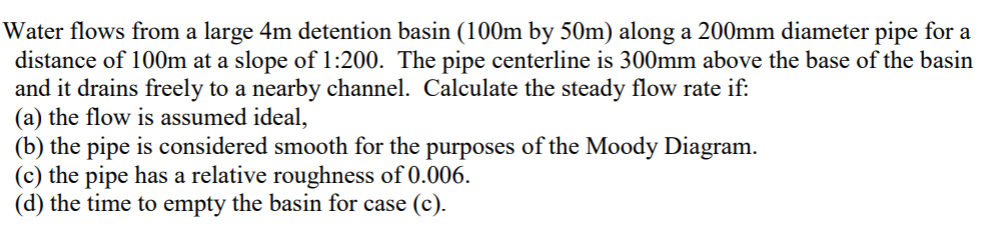Water flows from a large 4m detention basin (100m by 50m) along a 200mm diameter pipe for a distance of 100m at a slope of 1:200. The pipe centerline is 300mm above the base of the basin and it drains freely to a nearby channel. Calculate the steady flow rate if (a) the flow is assumed ideal, (b) the pipe is considered smooth for the purposes of the Moody Diagram (c) the pipe has a relative roughness of 0.006 (d) the time to empty the basin for case (c)

In this solution some basic concepts and formulas of Fluid Mechanics are used. For more information, refer to any standard textbook or drop a comment below. Please give a Thumbs Up, if solution is helpful.

Solution :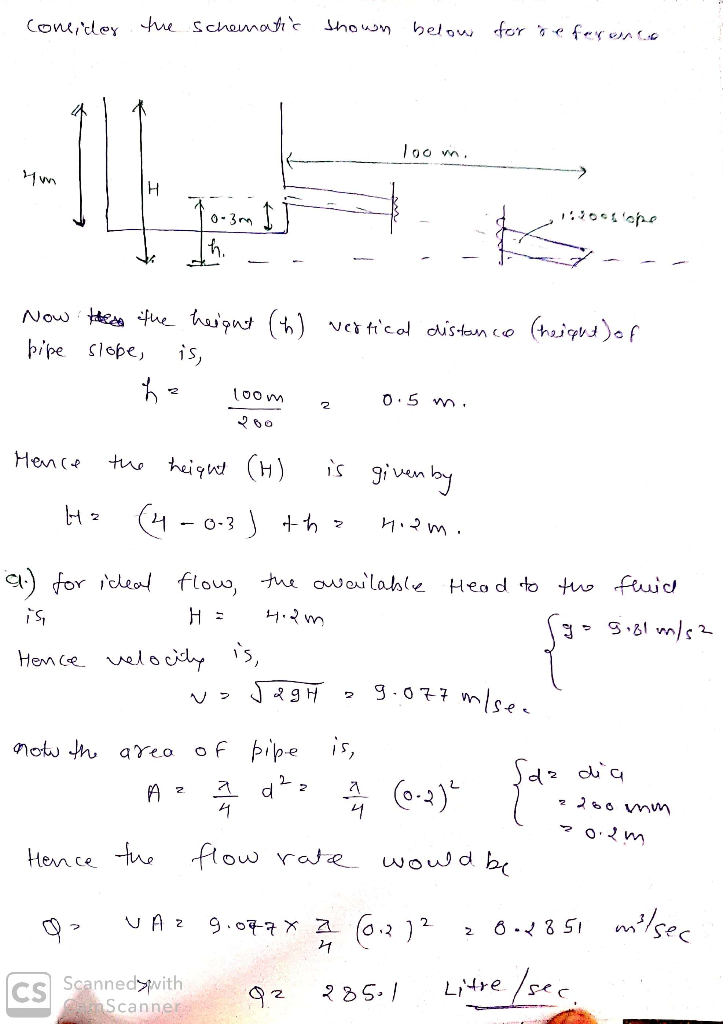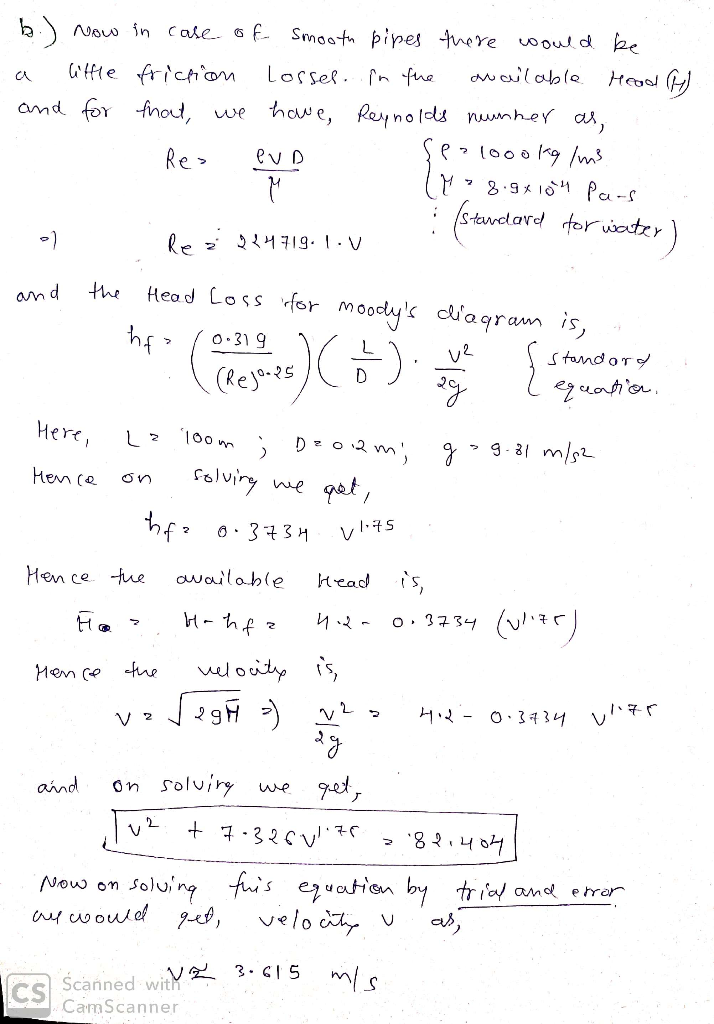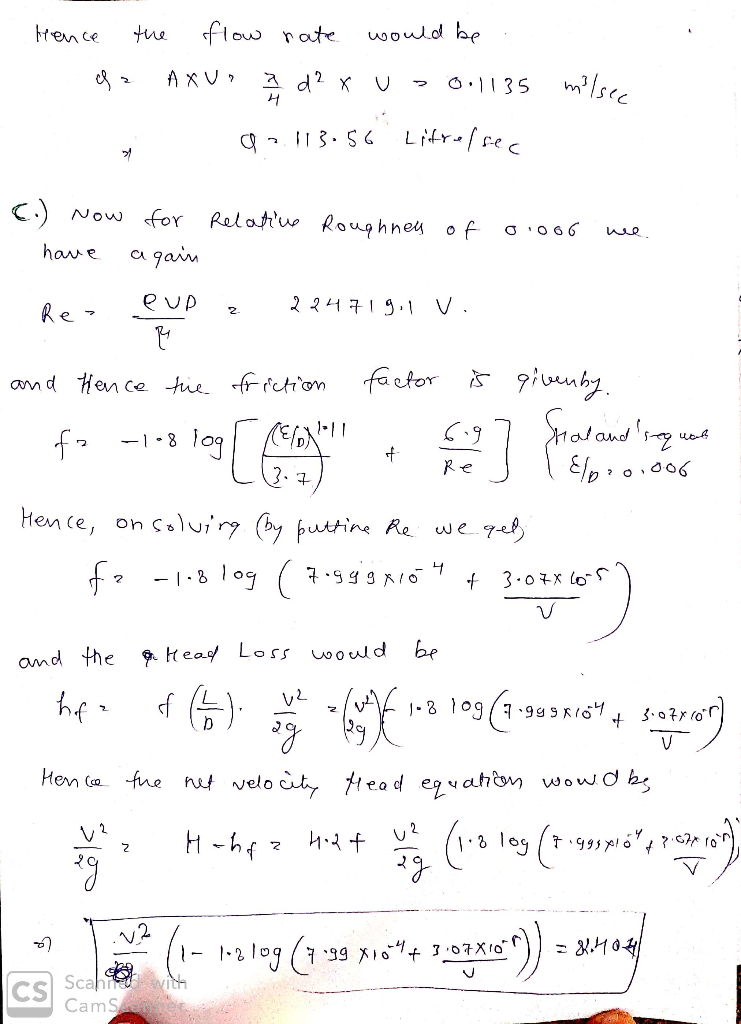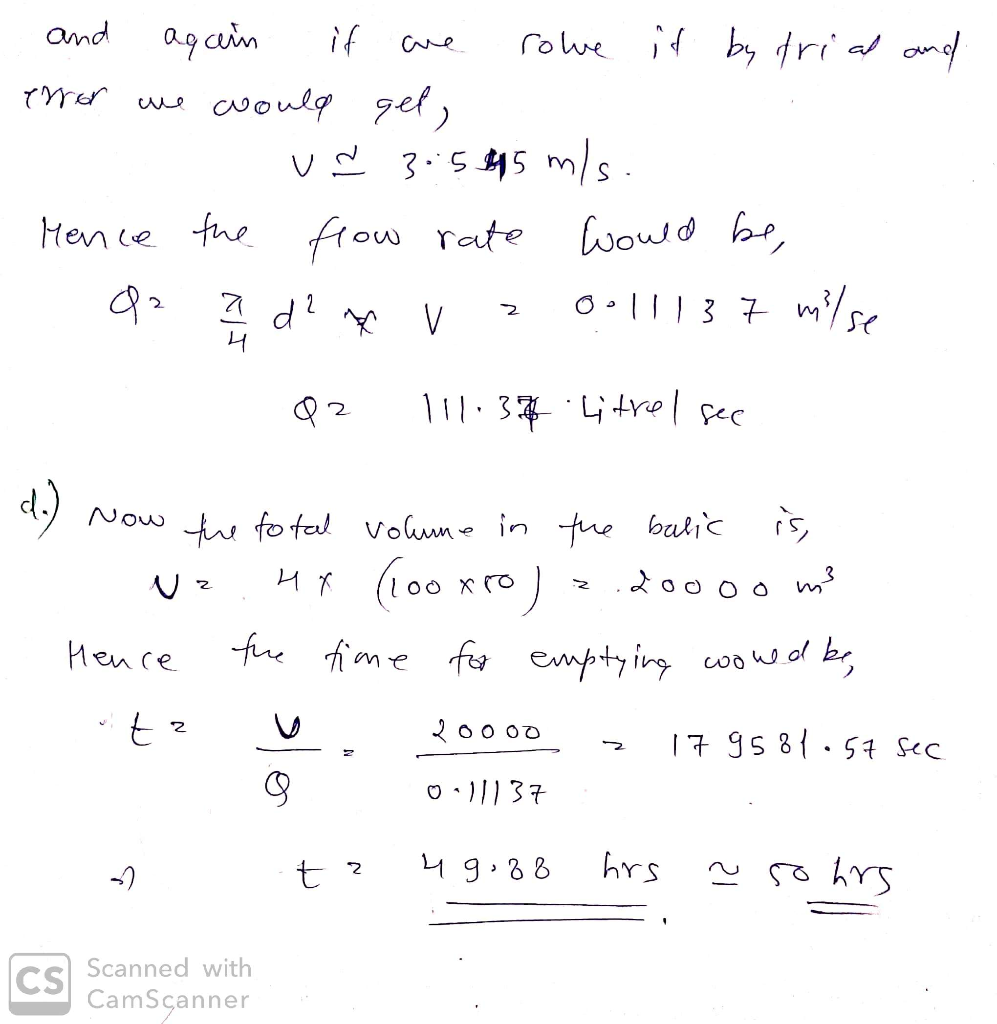NOTE : Feel free to ask further queries. Your positive rating would be appreciated and motivate me !

##### Add Answer of: Water flows from a large 4m detention basin (100m by 50m) along a 200mm diameter pipe for a distance of 100m at a slope...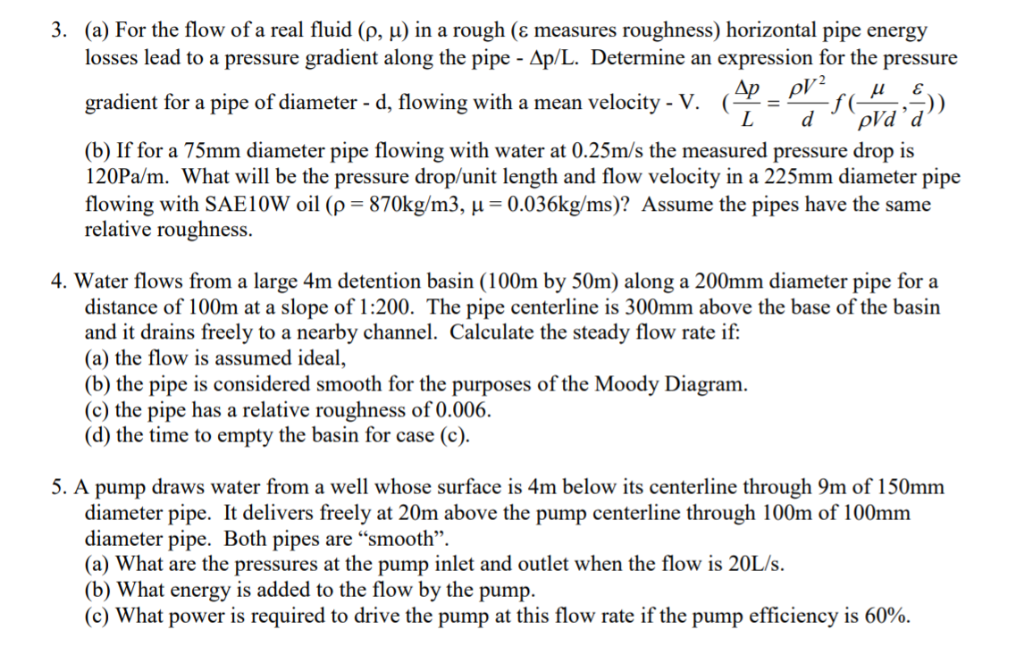3. (a) For the flow of a real fluid (p, u) in a rough (e measures losses lead to a pressure gradient along the pipe - Ap/L. Determine an expression for the pressure roughness) horizontal pipe energy Ap ( L pV2 gradient for a pipe of diameter - d, flowing with a mean velocity - V. pVd'd d (b) If for a 75mm diameter pipe flowing with water at 0.25m/s the measured pressure drop is 120Pa/m What will be the...